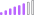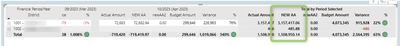cancel
Showing results for
Did you mean:Post Patron

## grand total incorrect when using an IF condition

Having issues with  getting matrix grand total correct when using an IF condition that if the district =1001, then sum the secondary currency else sum the local currency. Perhaps using selected value is incorrect.

NEW AA =
If(
SELECTEDVALUE('vw_districts'[District Code]) = "1001",
SUMX(vw_fin_transactions,'vw_fin_transactions'[Transaction Amount Secondary]),
SUMX(vw_fin_transactions,vw_fin_transactions[Transaction Amount Local])
)The model is pretty complex so I couldn't create a sample pbix. Hoping maybe someone has an idea of how to fix this.

1 ACCEPTED SOLUTIONPost Patron

I figured it out for anyone else dealing with this:

Actual_Amount =
SUMX(
vw_districts,
CALCULATE(
SWITCH(
MAX(vw_districts[District Code]),
"1001", SUM(vw_fin_transactions[Transaction Amount Secondary]),
SUM(vw_fin_transactions[Transaction Amount Local]))))
3 REPLIES 3Post Patron

I figured it out for anyone else dealing with this:

Actual_Amount =
SUMX(
vw_districts,
CALCULATE(
SWITCH(
MAX(vw_districts[District Code]),
"1001", SUM(vw_fin_transactions[Transaction Amount Secondary]),
SUM(vw_fin_transactions[Transaction Amount Local]))))Post Patron

thank you but I can't figure out how to write this with HASONEFILTER because the if statement after seems to me it's wanting to say if the sum is this, then use "this" else, use this measure.  (I underlined the part I am confused about)
I am not using a value so can't have if (sum) statement. What I need is clearly calling a table field and when I tried to add the condition If (district code = 1001...-it wouldn't let me use a table)
Mine is if the district code = 1001, then use the secondary currency, else use the local currency.

What works but totals are wrong.
Actual Amount =
If(
SELECTEDVALUE('vw_districts'[District Code]) = "1001",
SUMX(vw_fin_transactions,'vw_fin_transactions'[Transaction Amount Secondary]),
SUM(vw_fin_transactions[Transaction Amount Local])
)

This is what the references you sent were showing. I can't put an If condition calling a table field.
`MyMeasure2 = IF(HASONEFILTER(Table[Year]),IF(SUM(Table[Amount])<1000,0,SUM(Table[Amount])-1000),SUMX(FILTER(Table,[Amount]>1000),[Amount]-1000))`

Any thoughts on this?Super User

@electrobrit First, please vote for this idea: https://ideas.powerbi.com/ideas/idea/?ideaid=082203f1-594f-4ba7-ac87-bb91096c742e

This looks like a measure totals problem. Very common. See my post about it here: https://community.powerbi.com/t5/DAX-Commands-and-Tips/Dealing-with-Measure-Totals/td-p/63376

Also, this Quick Measure, Measure Totals, The Final Word should get you what you need:
https://community.powerbi.com/t5/Quick-Measures-Gallery/Measure-Totals-The-Final-Word/m-p/547907

Also: https://youtu.be/uXRriTN0cfY
And: https://youtu.be/n4TYhF2ARe8

Become an expert!: Enterprise DNA
External Tools: MSHGQM
Latest book!:
Mastering Power BI 2nd EditionDAX is easy, CALCULATE makes DAX hard...